Pandas is a a great package for data analysis. It is required by packages such as: xarray, seaborn, pymc3, plotnine, magenta, google-colab, featuretools, fastai, and others. In here we discuss how to load data into pandas dataframe.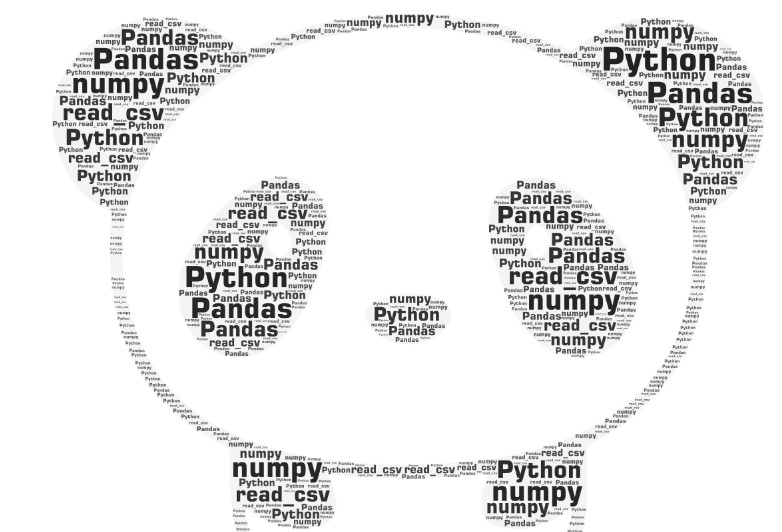## Creating a dataframe

Let’s create a dataframe in pandas. Simplified dataframe is a table. To create a table we just need the table size.

Example:

``````df = pd.DataFrame(index=range(14),columns=range(7))
print(df)
``````

Output: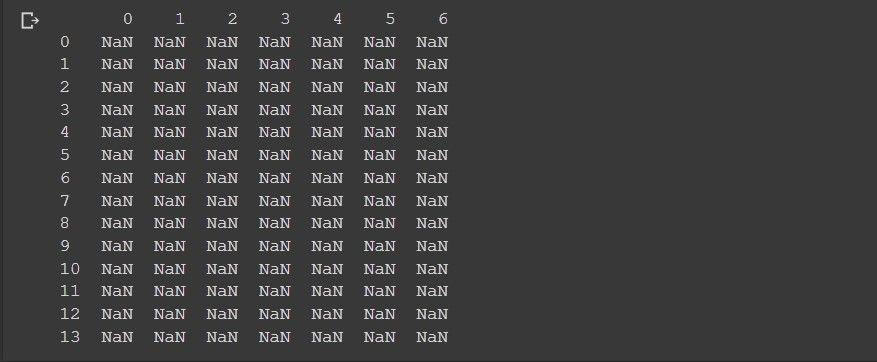Here we set the 7 columns and 14 rows. Note how in python pandas the start index is always 0.

To create a dataframe you may also provide the column names and row index names.

Example:

``````import pandas as pd
df = pd.DataFrame(index=['a', 'b', 'c'], columns=['time', 'date', 'name'])
print(df)
print(df.values)
``````

Output:

``````  time date name
a  NaN  NaN  NaN
b  NaN  NaN  NaN
c  NaN  NaN  NaN
[[nan nan nan]
[nan nan nan]
[nan nan nan]]
``````

From the output when we printed `print(df.values)` we got something that looks like a list. What is this?

Actually if we check the type of `type(df.values)` object we will find this is numpy.ndarray.

Pandas requires numpy, python-dateutil and pytz packages. Confirm that with `pip show pandas`.

Does this just mean we need numpy array to provide data to pandas dataframe?

No, because pandas is smart. Consider this example:

Example:

``````
data = pd.DataFrame([[10, 11, 12, 13, 14],
[22, 23, 24, 25, 26],
[31, 32, 33, 34, 35]])
print(data)
``````

Output:

``````    0   1   2   3   4
0  10  11  12  13  14
1  22  23  24  25  26
2  31  32  33  34  35
``````

In here we haven’t provided numpy arrays, instead the input data for the DataFrame is list of lists.

What will happen internally? Internally, pandas will convert the list of lists to numpy array.

Example:

``````import numpy as np
ll = [[10, 11, 12, 12, 14],
[22, 23, 21, 22, 23],
[31, 35, 32, 34, 34]]
npa = np.asarray(ll)
data = pd.DataFrame(npa)
print(data)
``````

Another example:

``````data = pd.DataFrame({'Col1': [10, 11, 12, 13, 14],
'Col2': [22, 23, 24, 25, 26],
'Col3': [31, 32, 33, 34, 35]})
print(data)
``````

Output:

``````   Col1  Col2  Col3
0    10    22    31
1    11    23    32
2    12    24    33
3    13    25    34
4    14    26    35
``````

If we plan to set the row names, this would be also possible:

Example:

``````data = pd.DataFrame({'Col1': [10, 11, 12, 13, 14],
'Col2': [22, 23, 24, 25, 26],
'Col3': [31, 32, 33, 34, 35]},
index=['row1', 'row2', 'row3', 'row4', 'row5'])
print(data)
``````

Output:

``````      Col1  Col2  Col3
row1    10    22    31
row2    11    23    32
row3    12    24    33
row4    13    25    34
row5    14    26    35
``````

### Comma separator

First example will use the `read_csv()` function to read a multiline text. CSV means Comma Separated Values. Using csv format is very frequent in pandas.

Example:

``````import pandas as pd
import io

text=u"""asdf,333
asdf,444
asdf,555"""

sep=r',',
engine='python',
encoding = "iso-8859-1")
print(df)
``````

Output:

``````      0    1
0  asdf  333
1  asdf  444
2  asdf  555
``````

Default `read_csv` separator is `sep=r','`.

### Space as separator

Let’s now use the same function but space as a separator.

``````import pandas as pd
import io

temp=u"""
NSW     VIC
6718023   5023203
6735528 5048207
6742690  5061266
6766133     5083593
6786160 5103965"""

sep=r'\s{1,}', # one or more spaces
engine='python',
encoding = "iso-8859-1")
print(df)
``````

Output:

``````       NSW      VIC
0  6718023  5023203
1  6735528  5048207
2  6742690  5061266
3  6766133  5083593
4  6786160  5103965
``````

### More than 1 spaces as separator

The next example will have NEW YORK as the index name, however, this should still be the single index name.

Example:

``````import pandas as pd
import io

s=u"""      one  two  three  four
INDIANA     0    1      2     3
NEW YORK    8    9     10    11
"""

sep=r'\s{2,}', # one or more spaces
engine='python',
encoding="iso-8859-1")
print(df)
``````

The solution here is to use two spaces as a separator.

Output:

``````          one  two  three  four
INDIANA     0    1      2     3
NEW YORK    8    9     10    11
``````

In here the dataframe index names are:

• INDIANA
• NEW YORK

### New line as a separator

Very similar example, but now, we use the new line as a separator.

Example:

``````from io import StringIO
import pandas as pd

text="""The lion (Panthera leo) is a species in the family Felidae.
It is a muscular, deep-chested cat with a short, rounded head.
A reduced neck and round ears, and a hairy tuft at the end of its tail.
The lion males are larger than females.
A typical weight range of 150 to 250 kg (330 to 550 lb) for males and 120 to 182 kg (265 to 400 lb) for females."""
sep=r'\n',
engine='python',
encoding = "iso-8859-1",
names=["cname"])
print(df)
print("size:", df.size)
``````

Output: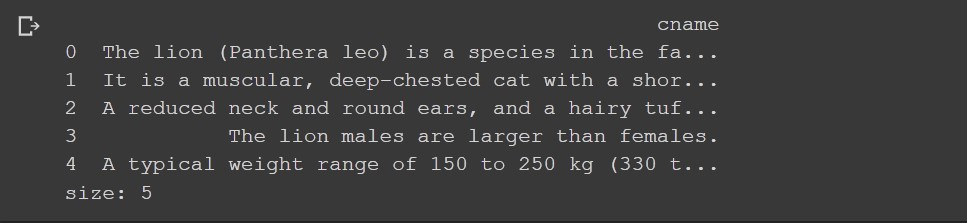One special option to read bad lines, would be `error_bad_lines=False`.

Example:

``````import pandas as pd
import io

s=u"""      one  two  three  four
INDIANA     0    1      2     3
NEW YORK    8    9     10    11
"""

sep=r'\s{1,}', # one or more spaces
engine='python',
encoding = "iso-8859-1")
print(df)
``````

Here the NEW YORK row will fail, because it has an extra column. If we use the `error_bad_lines=False` option we will ignore this problem.

Output:

``````          one  two  three  four
INDIANA     0    1      2     3

Skipping line 4: Expected 5 fields in line 4, saw 6. Error could possibly be due to quotes being ignored when a multi-char delimiter is used.
``````

## When the load is slow

Suppose you have 100 million rows inside your DataFrame. You may run out of memory unless you set the dtype parameter:

``````dtype: Type name or dict of column -> type, optional

Data type for data or columns. E.g. {'a': np.float64, 'b': np.int32, 'c': ‘Int64’} Use str or object together with suitable na_values settings to preserve and not interpret dtype. If converters are specified, they will be applied INSTEAD of dtype conversion.
``````

Because pandas will try to understand the column type by analyzing all the data inside any column.

In other words, by setting the column types within read_csv you will save some memory.

## Parse as a date type

When reading using read_csv method in case pandas cannot recognize the data type columns we may set the following:

``````df = pd.read_csv(f'{path}file.csv',
sep=r',',
parse_dates=['dc1', 'dc2'])
``````

In here the dc1 and dc2 are column names.

## Read dataframe from the CSV file

Another approach would be to read the CSV on disk file.

Again we will use `read_csv` function which is almost identical as the `read_table` function. The only difference is:

• `read_csv` uses comma separator
• `read_table` uses tab as separator

Usually the code to read the csv file will be short as this:

``````import pandas as pd
``````

First if there is no file you may get the `FileNotFoundError`. Frequent problem is the `UnicodeDecodeError`, where you should first understand the encoding of your file. To get the encoding of a file on Linux you may run `file -i` command and use that. If you think the things will work with the utf-8 encoding, you may use this trick:

• Read the text from a file
• Decode the text with `text.decode('utf-8')`

Usually utf-8 encoding, should cover the non-standard characters. If this doesn’t work try with the utf-16 encoding.

To fix the encoding problems you may use your editor and save the csv file to particular encoding.

In some cases you may get the parsing errors. If this is the case, use the engine=’python’ option.

## Read dataframe from remote csv file

Again, the same `read_csv` function works. This time we will use the `requests` package.

Check here -> how to use requests package.

Example:

``````import pandas as pd
import io
import requests
s=requests.get(url).content
``````

Output: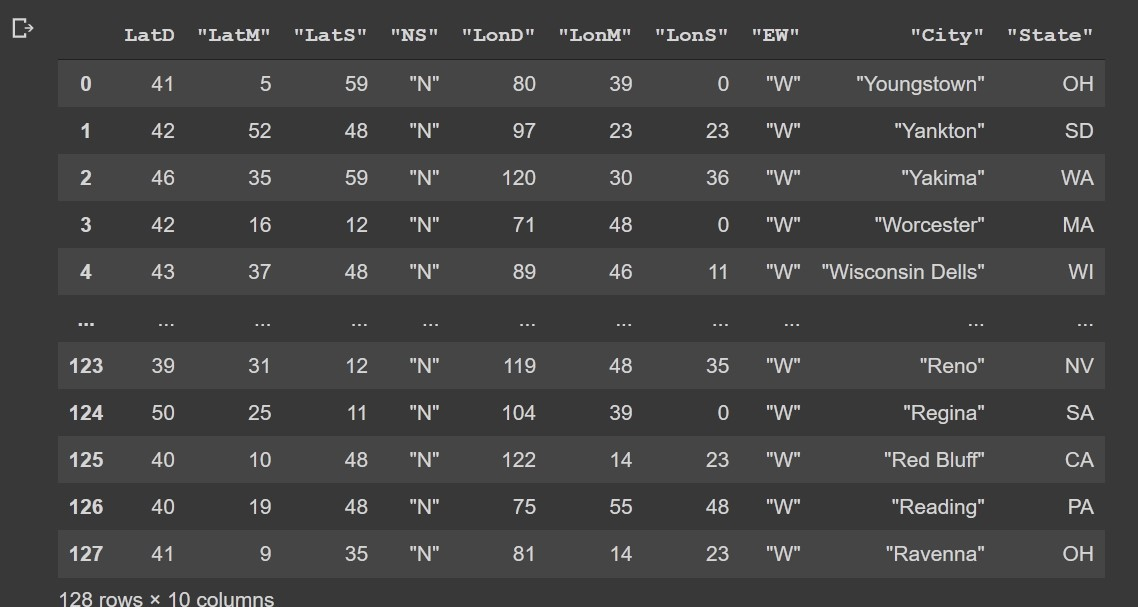## Read dataframe from HTML page

If there is a table (`<table>`) on a web page, pandas can read that. Note, this doesn’t work for divs.

Example:

``````import pandas as pd
df
``````

This is equivalent to:

``````url = 'https://en.wikipedia.org/wiki/Comparison_of_web_browsers'
html_data = requests.get(url)
df
``````

Output: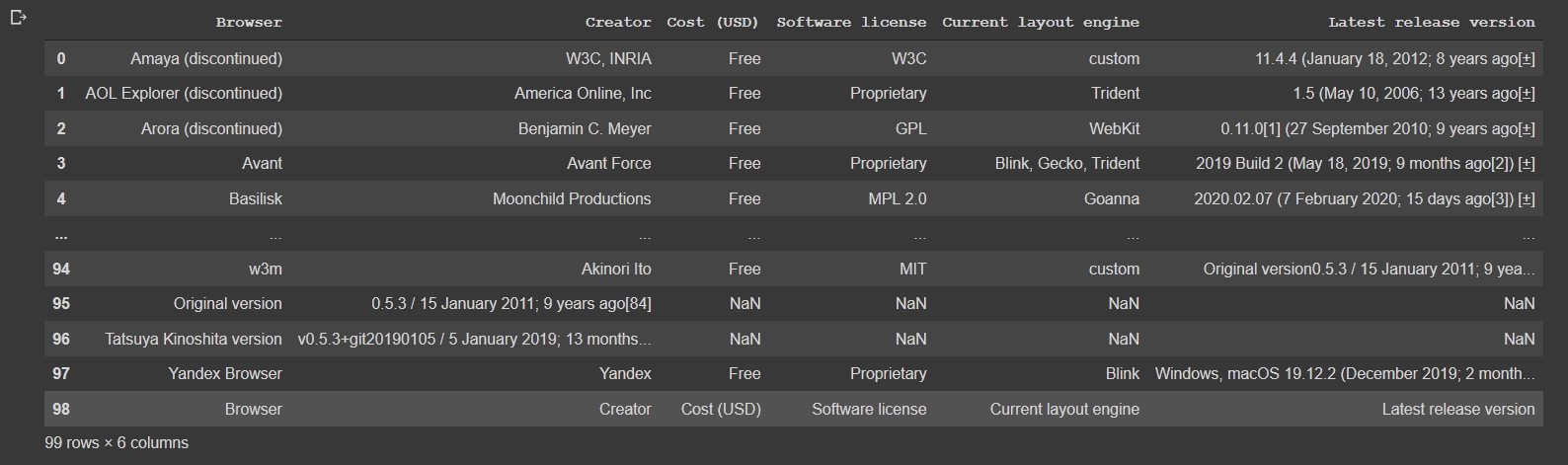## Fixing missing values

At times, you may have the missing values for some columns. It depends on a column, but often use this command:

``````df['col'].fillna((df['col'].median()), inplace=True)
``````

In here column col should be a numeric type. This can be done even more robust:

Example:

``````from pandas.api.types import is_string_dtype, is_numeric_dtype, is_categorical_dtype
import os
import numpy as np

df = pd.DataFrame({'col1' : [1, np.nan, 3], 'col2' : ['elementary school', 'high school', 'middle school']})

if is_numeric_dtype(df[cn]) and df[cn].isnull().sum():
df[cn+'_na']=df[cn].isnull()
df[cn].fillna(df[cn].median(), inplace=True)

df
``````

Output:

``````  col1  col2  col1_na
0   1.0   elementary school   False
1   2.0   high school   True
2   3.0   middle school   False
``````

Output:

``````  col1  col2
0   1.0   elementary school
1   2.0   high school
2   3.0   middle school
``````

While at first we had NaN special value.

``````  col1  col2
0   1.0   elementary school
1   NaN   high school
2   3.0   middle school
``````

## Convert string to categories

Often when we load data we like to convert string types to categories.

Example:

``````from pandas.api.types import is_string_dtype, is_numeric_dtype, is_categorical_dtype

df = pd.DataFrame({'col1' : [1, 2, 3], 'col2' : ['a', 'b', 'c']})

for k,v in df.items():
if is_string_dtype(v): df[k] = v.astype('category').cat.as_ordered()# as_unordered

df.col2.cat.categories
``````

Output:

``````#Index(['a', 'b', 'c'], dtype='object')
``````

With this process we converted the col2 column into type category from type object. df.info() yields:

``````<class 'pandas.core.frame.DataFrame'>
RangeIndex: 3 entries, 0 to 2
Data columns (total 2 columns):
col1    3 non-null int64
col2    3 non-null category
dtypes: category(1), int64(1)
memory usage: 259.0 bytes
``````

Before it was:

``````<class 'pandas.core.frame.DataFrame'>
RangeIndex: 3 entries, 0 to 2
Data columns (total 2 columns):
col1    3 non-null int64
col2    3 non-null object
dtypes: int64(1), object(1)
memory usage: 176.0+ bytes
``````

If you print the df you cannot change the difference, because the output will be the same in both the cases.

The change looks small, but beneath in pandas the difference is that we may now use machine learning algorithms on the non-string data.

To check some other forms of converting string data to numerical formats you may check the Encode Categorical Features article.

It is also possible to set your order of categories like this:

Example:

``````from pandas.api.types import is_string_dtype, is_numeric_dtype, is_categorical_dtype

df = pd.DataFrame({'col1' : [1, 2, 3], 'col2' : ['elementary school', 'high school', 'middle school']})
for k,v in df.items():
if is_string_dtype(v): df[k] = v.astype('category')

df.col2.cat.set_categories(['elementary school', 'middle school', 'high school'], ordered=True, inplace=True)
df.col2.cat.categories
``````

Output:

``````Index(['elementary school', 'middle school', 'high school'], dtype='object')
``````

Else the output would be alphabetical which is not what we may plan.

## What machine learning algorithms need?

What the machine learning algorithms do not expect are raw strings. The upper method of conversion to category astype(‘category’) will at the end provide code:

Example:

``````from pandas.api.types import is_string_dtype, is_numeric_dtype, is_categorical_dtype

df = pd.DataFrame({'col1' : [1, 2, 3], 'col2' : ['elementary school', 'high school', 'middle school']})
for k,v in df.items():
if is_string_dtype(v): df[k] = v.astype('category')

df.col2.cat.set_categories(['elementary school', 'middle school', 'high school'], ordered=True, inplace=True)
df.col2.cat.codes
``````

Output:

``````0    0
1    2
2    1
dtype: int8
``````

where in the column to the right 0 stands for elementary school, 1 stands for the middle school and 2 for high school.

## Fast save and load DataFrame in pandas

When dealing with big DataFrames (100M rows) you may find to_feather and read_feather as an excellent choice:

Example:

``````os.makedirs('tmp', exist_ok=True)
df.to_feather('tmp/row') # !pip install pyarrow
``````

To load the data you use:

Example:

``````df=pd.read_feather('tmp/row')
df
``````

This actually reads pandas memory and saves it to a disc and reads from disk to pandas memory.

tags: pandas - string - load & category: python